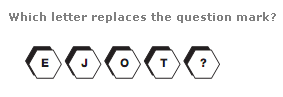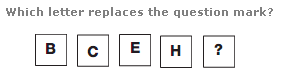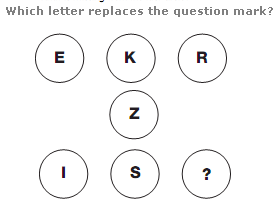# Puzzles - Missing letters puzzles

### Exercise :: Missing letters puzzlesAnswer : Y Explanation : From left to right, the numerical value of the letters represents the sequence of multiples of 5, from 5 to 25.Answer : M Explanation : Working downwards, add the numerical values of the first two letters together, and put the letter with this value in the next box down. Continue in this way down the diagram.Answer : D Explanation : Starting at the top left and moving in a Z shape around the diagram, the numerical value of the letters increases by 6, then 7, then 8 etc.